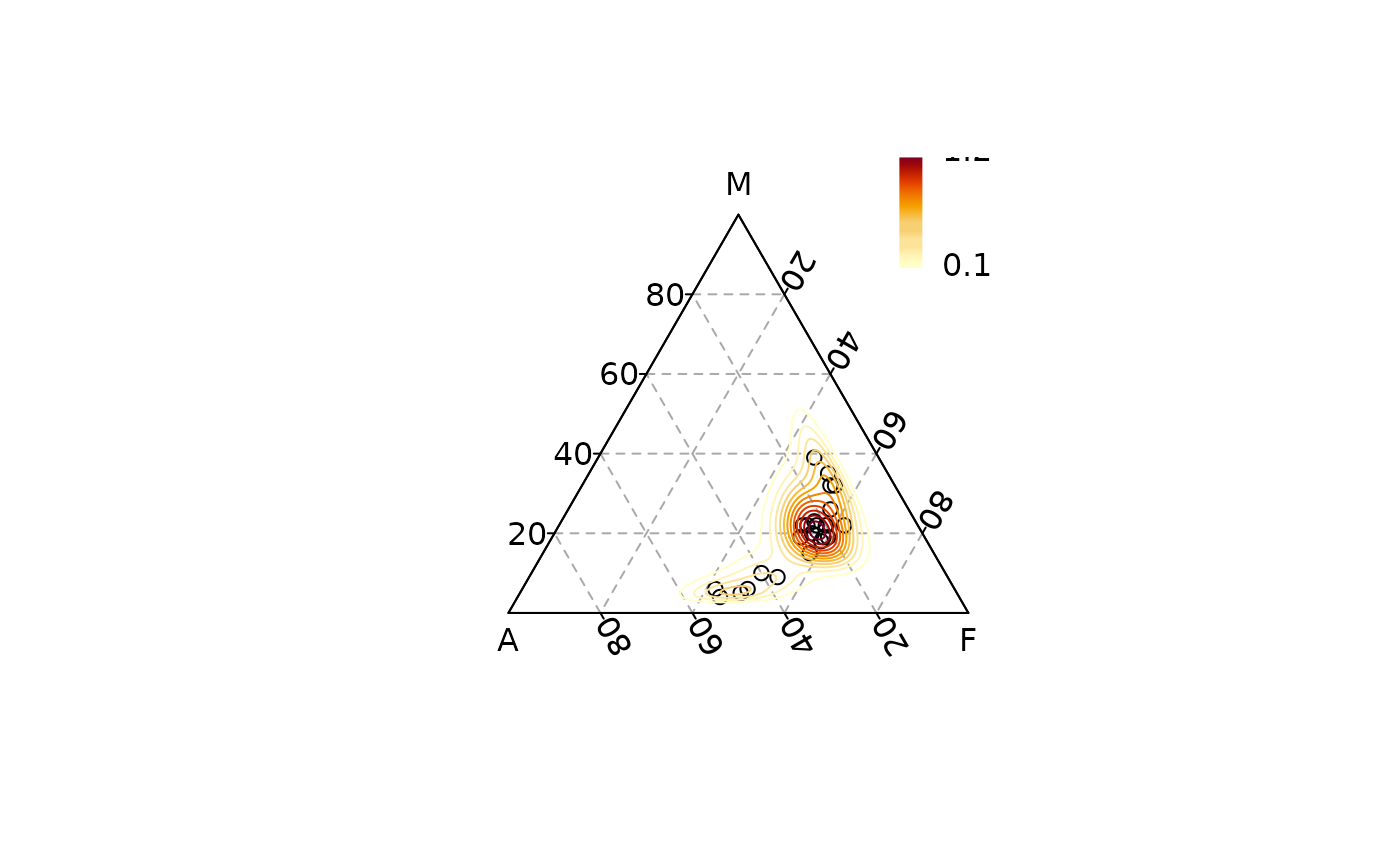Computes and draws density contour lines.

## Usage

ternary_density(x, y, z, ...)

# S4 method for numeric,numeric,numeric
ternary_density(
x,
y,
z,
h = NULL,
n = 25,
nlevels = 10,
levels = NULL,
palette = function(i) grDevices::hcl.colors(i, "YlOrRd", rev = TRUE),
...
)

# S4 method for ANY,missing,missing
ternary_density(
x,
h = NULL,
n = 25,
nlevels = 10,
levels = NULL,
palette = function(i) grDevices::hcl.colors(i, "YlOrRd", rev = TRUE),
...
)

## Arguments

x, y, z

A numeric vector giving the x, y and z ternary coordinates of a set of points. If y and z are missing, an attempt is made to interpret x in a suitable way (see grDevices::xyz.coords()).

...

Further arguments to be passed to ternary_lines().

h

A length-one numeric vector giving the bandwidth.

n

A length-one numeric specifying the number of grid points.

nlevels

A length-one numeric vector specifying the number of contour levels desired. Only used if levels is NULL.

levels

A numeric vector of levels at which to draw contour lines.

palette

A color palette function that takes a single integer argument (the number of levels) and returns a vector of colors.

## Value

ternary_density() is called it for its side-effects.

Invisibly returns a list with elements levels (the contour levels) and colors (the contour colors) that can be used for a legend.

## Details

Two-dimensional kernel density estimation with an axis-aligned bivariate normal kernel. Normal kernel is evaluated on a square grid, after an isometric log ratio transformation of the original data.

## Note

Two-dimensional kernel density estimation is adapted from MASS::kde2d().

This must be considered as experimental and subject to major changes in a future release.

grDevices::contourLines()

Other statistics: ternary_contour(), ternary_ellipse(), ternary_hull(), ternary_mean(), ternary_pca()

N. Frerebeau

## Examples

## Add density
## Data from Aitchison 1986
ternary_plot(lava, panel.first = ternary_grid())
levels <- ternary_density(lava, n = 500, nlevels = 10)

legend_image <- grDevices::as.raster(rev(levels$colors)) graphics::rasterImage(legend_image, 0.85, 0.75, 0.9, 1) graphics::text(x = 0.9, y = c(0.75, 1), labels = range(levels$levels), pos = 4)